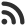Feed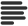Articles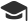Tutorials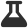CodingLab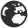CommunityLeaderboardFounder & Programmer at HackersFriend Updated Aug. 16, 2019, 7:41 p.m. ⋅ 223 views

# Sort an array without changing position of negative numbers

In this article I'll explain approach to sort an array that contains negative numbers, without changing the postion of negative numbers. i.e We'll have to ignore negative numbers.

Problem

You are given an aray arr [ ] that contains, N integers. They are negative and non negative. Task is to leave negative numbers as it is and sort rest of the elements of array.

Example

Input

``arr[] = {2, -7, -19, 8, 4, 1}``

Output

``1 -7 -19 2 4 8``

Explanation

Here, you can see, we have left negative numbers -7 and -19 as their place and sorted other numbers.

Solution

Idea to solve this problem is to take all non negative numbers into a seperate vector and sort this vector. Then, replace all non-negative numbers of aaray with these sorted numbers.

Let's code ths approach.

``````#include <bits/stdc++.h>
using namespace std;

void sortArray(int a[], int n)
{

// Storing all non-negative values
vector<int> ans;
for (int i = 0; i < n; i++) {
if (a[i] >= 0)
ans.push_back(a[i]);
}

// Sorting non-negative values
sort(ans.begin(), ans.end());

int j = 0;
for (int i = 0; i < n; i++) {

// If current element is non-negative then
// update it such that all the
// non-negative values are sorted
if (a[i] >= 0) {
a[i] = ans[j];
j++;
}
}

// Print the sorted array
for (int i = 0; i < n; i++)
cout << a[i] << " ";
}

// Main functipm
int main()
{
int arr[] = { 2, -7, -19, 8, 4, 1 };

int n = sizeof(arr) / sizeof(arr);

sortArray(arr, n);

return 0;
}
``````

Output

``1 -7 -19 2 4 8``

 arrow_upward UpvoteComment arrow_downward downvote

Events

Nov. 28, 2018, 5:30 p.m.

Python from zero to hero

place Delhi

Aug. 13, 2018, 5:30 p.m.

Python from zero to hero

place Bangalore ( HackersFriend office BTM Layout)